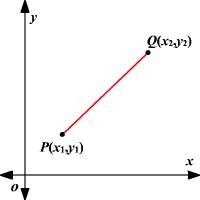# Magnitude and Direction of Vectors

### Magnitude of a Vector

The magnitude of a vector $\stackrel{\to }{PQ}$ is the distance between the initial point $P$ and the end point $Q$ . In symbols the magnitude of $\stackrel{\to }{PQ}$ is written as $|\text{\hspace{0.17em}}\stackrel{\to }{PQ}\text{\hspace{0.17em}}|$ .

If the coordinates of the initial point and the end point of a vector is given, the Distance Formula can be used to find its magnitude.$|\text{\hspace{0.17em}}\stackrel{\to }{PQ}\text{\hspace{0.17em}}|=\sqrt{{\left({x}_{2}-{x}_{1}\right)}^{2}+{\left({y}_{2}-{y}_{1}\right)}^{2}}$

Example 1:

Find the magnitude of the vector $\stackrel{\to }{PQ}$ whose initial point $P$ is at $\left(1,1\right)$ and end point is at $Q$ is at $\left(5,3\right)$ .

Solution:

Use the Distance Formula.

Substitute the values of ${x}_{1}$ , ${y}_{1}$ , ${x}_{2}$ , and ${y}_{2}$ .

$\begin{array}{l}|\text{\hspace{0.17em}}\stackrel{\to }{PQ}\text{\hspace{0.17em}}|=\sqrt{{\left(5-1\right)}^{2}+{\left(3-1\right)}^{2}}\\ \text{\hspace{0.17em}}\text{\hspace{0.17em}}\text{\hspace{0.17em}}\text{\hspace{0.17em}}\text{\hspace{0.17em}}\text{\hspace{0.17em}}\text{\hspace{0.17em}}\text{\hspace{0.17em}}\text{\hspace{0.17em}}\text{\hspace{0.17em}}\text{\hspace{0.17em}}=\sqrt{{4}^{2}+{2}^{2}}\\ \text{\hspace{0.17em}}\text{\hspace{0.17em}}\text{\hspace{0.17em}}\text{\hspace{0.17em}}\text{\hspace{0.17em}}\text{\hspace{0.17em}}\text{\hspace{0.17em}}\text{\hspace{0.17em}}\text{\hspace{0.17em}}\text{\hspace{0.17em}}\text{\hspace{0.17em}}=\sqrt{16+4}\\ \text{\hspace{0.17em}}\text{\hspace{0.17em}}\text{\hspace{0.17em}}\text{\hspace{0.17em}}\text{\hspace{0.17em}}\text{\hspace{0.17em}}\text{\hspace{0.17em}}\text{\hspace{0.17em}}\text{\hspace{0.17em}}\text{\hspace{0.17em}}\text{\hspace{0.17em}}=\sqrt{20}\\ \text{\hspace{0.17em}}\text{\hspace{0.17em}}\text{\hspace{0.17em}}\text{\hspace{0.17em}}\text{\hspace{0.17em}}\text{\hspace{0.17em}}\text{\hspace{0.17em}}\text{\hspace{0.17em}}\text{\hspace{0.17em}}\text{\hspace{0.17em}}\text{\hspace{0.17em}}\approx 4.5\end{array}$

The magnitude of $\stackrel{\to }{PQ}$ is about $4.5$ .

### Direction of a Vector

The direction of a vector is the measure of the angle it makes with a horizontal line .

One of the following formulas can be used to find the direction of a vector:

$\mathrm{tan}\theta =\frac{y}{x}$ , where $x$ is the horizontal change and $y$ is the vertical change

or

$\mathrm{tan}\theta =\frac{{y}_{2}\text{\hspace{0.17em}}-\text{\hspace{0.17em}}{y}_{1}}{{x}_{2}\text{\hspace{0.17em}}-\text{\hspace{0.17em}}{x}_{1}}$ , where $\left({x}_{1},{y}_{1}\right)$ is the initial point and $\left({x}_{2},{y}_{2}\right)$ is the terminal point.

Example 2:

Find the direction of the vector $\stackrel{\to }{PQ}$ whose initial point $P$ is at $\left(2,3\right)$ and end point is at $Q$ is at $\left(5,8\right)$ .

The coordinates of the initial point and the terminal point are given. Substitute them in the formula $\mathrm{tan}\theta =\frac{{y}_{2}\text{\hspace{0.17em}}-\text{\hspace{0.17em}}{y}_{1}}{{x}_{2}\text{\hspace{0.17em}}-\text{\hspace{0.17em}}{x}_{1}}$ .

$\begin{array}{l}\mathrm{tan}\theta =\frac{8\text{\hspace{0.17em}}-\text{\hspace{0.17em}}3}{5\text{\hspace{0.17em}}-\text{\hspace{0.17em}}2}\\ \text{\hspace{0.17em}}\text{\hspace{0.17em}}\text{\hspace{0.17em}}\text{\hspace{0.17em}}\text{\hspace{0.17em}}\text{\hspace{0.17em}}\text{\hspace{0.17em}}\text{\hspace{0.17em}}\text{\hspace{0.17em}}\text{\hspace{0.17em}}\text{\hspace{0.17em}}\text{\hspace{0.17em}}=\frac{5}{3}\end{array}$

Find the inverse tan, then use a calculator.

$\begin{array}{l}\theta ={\mathrm{tan}}^{-1}\left(\frac{5}{3}\right)\\ \text{\hspace{0.17em}}\text{\hspace{0.17em}}\text{\hspace{0.17em}}\text{\hspace{0.17em}}\approx 59°\end{array}$

The vector $\stackrel{\to }{PQ}$ has a direction of about $59°$ .